Courses

# Test: Nitrogen & Phosphorous Compounds

## 20 Questions MCQ Test Chemistry Class 12 | Test: Nitrogen & Phosphorous Compounds

Description
This mock test of Test: Nitrogen & Phosphorous Compounds for JEE helps you for every JEE entrance exam. This contains 20 Multiple Choice Questions for JEE Test: Nitrogen & Phosphorous Compounds (mcq) to study with solutions a complete question bank. The solved questions answers in this Test: Nitrogen & Phosphorous Compounds quiz give you a good mix of easy questions and tough questions. JEE students definitely take this Test: Nitrogen & Phosphorous Compounds exercise for a better result in the exam. You can find other Test: Nitrogen & Phosphorous Compounds extra questions, long questions & short questions for JEE on EduRev as well by searching above.
QUESTION: 1

### Direction (Q. Nos. 1-7) This section contains 7 multiple choice questions. Each question has four choices (a), (b), (c) and (d), out of which ONLY ONE is correct. Q.  The hybridisation of N in solid state for N2O5 is

Solution:

In solid state, N2O5 exists as nitronium nitrate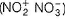In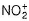nitrogen is sp and in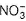, nitrogen is sp2.

QUESTION: 2

### PH3 becomes spontaneously inflammable due to the presence of

Solution:

Pure phoshine is not inflammable but due to contamination of P2H4 and P4 vapours, it becomes inflammabl.

QUESTION: 3

### Which is incorrect among the following options?

Solution:

Reducing property of P oxyacids is due to P—H bonds in the acid. Correct reducing property order is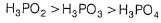QUESTION: 4

The number of P – O bonds and lone pairs of electron present in P4O6 molecule respectively

Solution: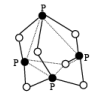Number of P – O bonds = 12
Number of pair of electron = 16

QUESTION: 5

Which is the correct order of basic strength ?

Solution:

NH2OH and NH2—NH2 may be considered as NH3 derivatives in which H is replaced but — OH and NH2 respectively. Due to their electron withdrawing nature, these groups decreases electron density over nitrogen making them less basic. The effect of — OH group is stronger than —NH2

QUESTION: 6

Solid PCl5 and solid PBr5 exist respectively as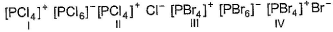Solution: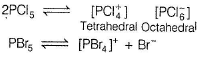PBr does not split in the same fashion. The anion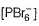is not possible due to large size of Br-atoms. Hence, it splits differently.

QUESTION: 7

Number of moles of NaOH needed to neutralise one mole each of H3PO2, H3PO3 and H3PO4 respectively are

Solution:

H3PO2, H3PO3 and H3PO4 are mono, di and tribasic acids respectively.

*Multiple options can be correct
QUESTION: 8

Direction (Q. Nos. 8-12) This section contains 5 multiple choice questions. Each question has four choices (a), (b), (c) and (d), out of which ONE or MORE THAN ONE are correct.

Q.

Which of the following can act as dehydrating agent ?

Solution:

P4O10,POCI3 and cone. H2SO4 are act as dehydrating agent.

*Multiple options can be correct
QUESTION: 9

NO2 is obtained by heating

Solution:

Sodium and potassium nitrate on heating gives nitrite and oxygen but not NO2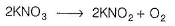*Multiple options can be correct
QUESTION: 10

Phosphine is obtained when

Solution: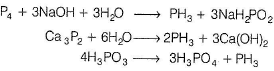*Multiple options can be correct
QUESTION: 11

Regarding ammonia correct statements are

Solution:

Metal nitride upon hydrolysis gives ammonia,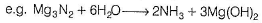As we know Haber's process involves the direct combination of nitrogen and hydrogen with the removal of some amount of heat, i.e. this is exothermic in nature.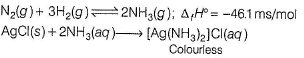Nitrogen atom of ammonia can donate a lone pair of e- to any species that is why, Lewis base as well as Bronsted-Lowry base.

*Multiple options can be correct
QUESTION: 12

Which is correctly matched?

Solution: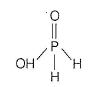Since, here 1-hydroxyl group is present so, it is monobasic acid. Since, metaphosphoric, when reacts in the presence of heat orthophosphoric acid is form which is in the form of glacial phosphoric acid.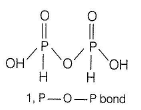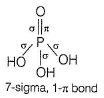- H4P2O5 (phosphorus acid) has P— O—P bond
Option (a) and (c) is not correctly matched.

QUESTION: 13

Direction (Q. Nos. 13 and 14) This section contains a paragraph, describing theory, experiments, data, etc. Two questions related to the paragraph have been given. Each question has only one correct answer among the four given options (a), (b), (c) and (d).

Passage

In the all oxyacids of phosphorus, each phosphorus atom is in sp3-hybridised state. All these acids contain P—OH bonds, the hydrogen atom of which are ionisable imparting acidic nature to the compound. The ‘ous’ acids (oxidation state of P is + 1 or + 3) also have P—H bonds in which hydrogens are not ionisable.
The presence of P—H bonds in these acids imparts reducing properties. The structure of some oxyacids are drawn below: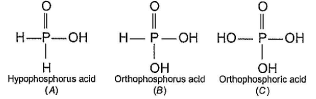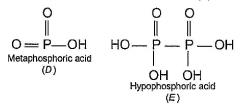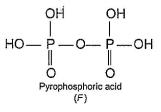Q.

Although metaphosphoric acid is written as a monomer, it exists as a polymer (HPO3)n. The number of P—O—P bonds in cyclic trimetaphosphoric acid is

Solution: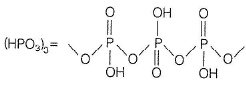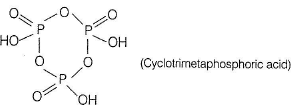QUESTION: 14

In the all oxyacids of phosphorus, each phosphorus atom is in sp3-hybridised state. All these acids contain P—OH bonds, the hydrogen atom of which are ionisable imparting acidic nature to the compound. The ‘ous’ acids (oxidation state of P is + 1 or + 3) also have P—H bonds in which hydrogens are not ionisable.
The presence of P—H bonds in these acids imparts reducing properties. The structure of some oxyacids are drawn below:Q.

Which of the acids show reducing properties?

Solution:

Due to the presence of H in A and B , they showre ducing properties.

QUESTION: 15

Direction (Q. Nos. 15 and 16) Choices for the correct combination of elements from Column I and Column II are given as options (a), (b), (c) and (d), out of which one is correct.

Q.

Match the Column I with Column II and mark the correct option from the codes given below.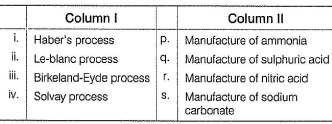Solution: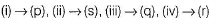QUESTION: 16

Match the Column I with Column II and mark the correct option from the codes given below.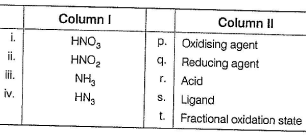Solution: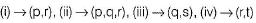*Answer can only contain numeric values
QUESTION: 17

Direction (Q. Nos. 17-19) This section contains 3 questions. When worked out will result in an integer from 0 to 9 (both inclusive).

Q.

Number of chemical species having bond between nitrogen atoms

N2O4, N2O5, N2H4, N2O , N2O3, NH4NO3, H2N2O2

Solution:

N2O5 , N2O and NH4NO3 have no bond between nitrogen atoms while others have.

*Answer can only contain numeric values
QUESTION: 18

Number of metals that show passivity with cone. HNO3 among Cr, Fe, Ni, Cu, Zn Al, Ag, Sn.

Solution:

Cr, Fe, Ni and Al are passive towards cone . HNO3.

*Answer can only contain numeric values
QUESTION: 19

2H3PO2 → H3PO4 + PH3  in this equivalent weight of the acid in the reactant side is obtained by dividing molecular weight with

Solution: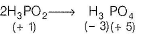Equivalent weight, E1 (oxidation) = M/4 and
Equivalent weight, E2 (reduction)
= M /4
Equivalent wight of H3PO2 in the disproportionation reaction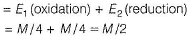Molecular nitrogen is less reactive than that of oxygen because nitrogen has high dissociation energy in comparison to oxygen so, its reactivity is less. Also bond length of nitrogen is shorter than oxygen because of the presence of triple bond between nitrogen atoms. So, both are true but not correct explanation.

QUESTION: 20

Direction (Q. No. 20)  This section is bassed on Statment I and Statment II. II. Select the correct answer from the codes given below.

Q.

Statement I : NF3 is a weaker ligand than N(CH3)3

Statement II : NF3 ionises to give F- ions in aqueous solutio

Solution:

Due to e- withdrawing capacity of fluorideion, it withdraws. from nitrogen in NF3 make it weakerlig and while presence of e- donating methyl group makes the nitrogen in N(CH3)3 a strong ligand. In aqueous medium, NF3 furnishes fluorideion.